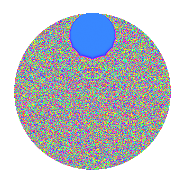# Properties

 Label 2.82.a.aLevel 2 Weight 82 Character orbit 2.a Self dual Yes Analytic conductor 83.100 Analytic rank 1 Dimension 3 CM No Inner twists 1

# Related objects

## Newspace parameters

 Level: $$N$$ = $$2$$ Weight: $$k$$ = $$82$$ Character orbit: $$[\chi]$$ = 2.a (trivial)

## Newform invariants

 Self dual: Yes Analytic conductor: $$83.1002571076$$ Analytic rank: $$1$$ Dimension: $$3$$ Coefficient field: $$\mathbb{Q}[x]/(x^{3} - \cdots)$$ Coefficient ring: $$\Z[a_1, \ldots, a_{5}]$$ Coefficient ring index: $$2^{23}\cdot 3^{10}\cdot 5^{3}\cdot 7$$ Fricke sign: $$1$$ Sato-Tate group: $\mathrm{SU}(2)$

## $q$-expansion

Coefficients of the $$q$$-expansion are expressed in terms of a basis $$1,\beta_1,\beta_2$$ for the coefficient ring described below. We also show the integral $$q$$-expansion of the trace form.

 $$f(q)$$ $$=$$ $$q -1099511627776 q^{2} +(4201453557463404996 + \beta_{1}) q^{3} +$$$$12\!\cdots\!76$$$$q^{4} +(-$$$$69\!\cdots\!50$$$$+ 298982601 \beta_{1} + 769 \beta_{2}) q^{5} +(-$$$$46\!\cdots\!96$$$$- 1099511627776 \beta_{1}) q^{6} +(-$$$$18\!\cdots\!08$$$$- 446074228826442 \beta_{1} + 572763764 \beta_{2}) q^{7} -$$$$13\!\cdots\!76$$$$q^{8} +(-$$$$39\!\cdots\!87$$$$+ 4707282525607846350 \beta_{1} + 9842345463582 \beta_{2}) q^{9} +O(q^{10})$$ $$q -1099511627776 q^{2} +(4201453557463404996 + \beta_{1}) q^{3} +$$$$12\!\cdots\!76$$$$q^{4} +(-$$$$69\!\cdots\!50$$$$+ 298982601 \beta_{1} + 769 \beta_{2}) q^{5} +(-$$$$46\!\cdots\!96$$$$- 1099511627776 \beta_{1}) q^{6} +(-$$$$18\!\cdots\!08$$$$- 446074228826442 \beta_{1} + 572763764 \beta_{2}) q^{7} -$$$$13\!\cdots\!76$$$$q^{8} +(-$$$$39\!\cdots\!87$$$$+ 4707282525607846350 \beta_{1} + 9842345463582 \beta_{2}) q^{9} +($$$$76\!\cdots\!00$$$$-$$$$32\!\cdots\!76$$$$\beta_{1} - 845524441759744 \beta_{2}) q^{10} +($$$$42\!\cdots\!12$$$$+$$$$24\!\cdots\!39$$$$\beta_{1} - 23868188313924152 \beta_{2}) q^{11} +($$$$50\!\cdots\!96$$$$+$$$$12\!\cdots\!76$$$$\beta_{1}) q^{12} +(-$$$$67\!\cdots\!14$$$$+$$$$32\!\cdots\!61$$$$\beta_{1} - 34303936689160258679 \beta_{2}) q^{13} +($$$$20\!\cdots\!08$$$$+$$$$49\!\cdots\!92$$$$\beta_{1} -$$$$62\!\cdots\!64$$$$\beta_{2}) q^{14} +($$$$86\!\cdots\!00$$$$+$$$$71\!\cdots\!66$$$$\beta_{1} +$$$$90\!\cdots\!04$$$$\beta_{2}) q^{15} +$$$$14\!\cdots\!76$$$$q^{16} +($$$$45\!\cdots\!82$$$$-$$$$71\!\cdots\!54$$$$\beta_{1} -$$$$29\!\cdots\!58$$$$\beta_{2}) q^{17} +($$$$43\!\cdots\!12$$$$-$$$$51\!\cdots\!00$$$$\beta_{1} -$$$$10\!\cdots\!32$$$$\beta_{2}) q^{18} +($$$$15\!\cdots\!20$$$$-$$$$20\!\cdots\!75$$$$\beta_{1} -$$$$19\!\cdots\!68$$$$\beta_{2}) q^{19} +(-$$$$84\!\cdots\!00$$$$+$$$$36\!\cdots\!76$$$$\beta_{1} +$$$$92\!\cdots\!44$$$$\beta_{2}) q^{20} +(-$$$$17\!\cdots\!68$$$$+$$$$10\!\cdots\!96$$$$\beta_{1} +$$$$13\!\cdots\!88$$$$\beta_{2}) q^{21} +(-$$$$46\!\cdots\!12$$$$-$$$$26\!\cdots\!64$$$$\beta_{1} +$$$$26\!\cdots\!52$$$$\beta_{2}) q^{22} +(-$$$$10\!\cdots\!24$$$$-$$$$21\!\cdots\!18$$$$\beta_{1} +$$$$20\!\cdots\!44$$$$\beta_{2}) q^{23} +(-$$$$55\!\cdots\!96$$$$-$$$$13\!\cdots\!76$$$$\beta_{1}) q^{24} +($$$$93\!\cdots\!75$$$$+$$$$79\!\cdots\!00$$$$\beta_{1} -$$$$18\!\cdots\!00$$$$\beta_{2}) q^{25} +($$$$74\!\cdots\!64$$$$-$$$$35\!\cdots\!36$$$$\beta_{1} +$$$$37\!\cdots\!04$$$$\beta_{2}) q^{26} +(-$$$$20\!\cdots\!40$$$$-$$$$30\!\cdots\!54$$$$\beta_{1} +$$$$12\!\cdots\!16$$$$\beta_{2}) q^{27} +(-$$$$22\!\cdots\!08$$$$-$$$$53\!\cdots\!92$$$$\beta_{1} +$$$$69\!\cdots\!64$$$$\beta_{2}) q^{28} +($$$$71\!\cdots\!30$$$$-$$$$48\!\cdots\!03$$$$\beta_{1} -$$$$34\!\cdots\!43$$$$\beta_{2}) q^{29} +(-$$$$94\!\cdots\!00$$$$-$$$$79\!\cdots\!16$$$$\beta_{1} -$$$$99\!\cdots\!04$$$$\beta_{2}) q^{30} +(-$$$$12\!\cdots\!68$$$$-$$$$19\!\cdots\!64$$$$\beta_{1} +$$$$37\!\cdots\!48$$$$\beta_{2}) q^{31} -$$$$16\!\cdots\!76$$$$q^{32} +($$$$27\!\cdots\!52$$$$-$$$$74\!\cdots\!78$$$$\beta_{1} -$$$$16\!\cdots\!78$$$$\beta_{2}) q^{33} +(-$$$$50\!\cdots\!32$$$$+$$$$78\!\cdots\!04$$$$\beta_{1} +$$$$32\!\cdots\!08$$$$\beta_{2}) q^{34} +($$$$26\!\cdots\!00$$$$+$$$$34\!\cdots\!32$$$$\beta_{1} -$$$$14\!\cdots\!92$$$$\beta_{2}) q^{35} +(-$$$$47\!\cdots\!12$$$$+$$$$56\!\cdots\!00$$$$\beta_{1} +$$$$11\!\cdots\!32$$$$\beta_{2}) q^{36} +(-$$$$32\!\cdots\!38$$$$+$$$$13\!\cdots\!21$$$$\beta_{1} -$$$$23\!\cdots\!03$$$$\beta_{2}) q^{37} +(-$$$$17\!\cdots\!20$$$$+$$$$22\!\cdots\!00$$$$\beta_{1} +$$$$21\!\cdots\!68$$$$\beta_{2}) q^{38} +($$$$12\!\cdots\!56$$$$-$$$$67\!\cdots\!62$$$$\beta_{1} +$$$$45\!\cdots\!00$$$$\beta_{2}) q^{39} +($$$$92\!\cdots\!00$$$$-$$$$39\!\cdots\!76$$$$\beta_{1} -$$$$10\!\cdots\!44$$$$\beta_{2}) q^{40} +($$$$10\!\cdots\!42$$$$-$$$$75\!\cdots\!00$$$$\beta_{1} -$$$$31\!\cdots\!04$$$$\beta_{2}) q^{41} +($$$$19\!\cdots\!68$$$$-$$$$11\!\cdots\!96$$$$\beta_{1} -$$$$14\!\cdots\!88$$$$\beta_{2}) q^{42} +($$$$30\!\cdots\!56$$$$+$$$$28\!\cdots\!99$$$$\beta_{1} +$$$$53\!\cdots\!04$$$$\beta_{2}) q^{43} +($$$$51\!\cdots\!12$$$$+$$$$29\!\cdots\!64$$$$\beta_{1} -$$$$28\!\cdots\!52$$$$\beta_{2}) q^{44} +($$$$62\!\cdots\!50$$$$+$$$$12\!\cdots\!53$$$$\beta_{1} -$$$$19\!\cdots\!43$$$$\beta_{2}) q^{45} +($$$$11\!\cdots\!24$$$$+$$$$24\!\cdots\!68$$$$\beta_{1} -$$$$22\!\cdots\!44$$$$\beta_{2}) q^{46} +($$$$54\!\cdots\!52$$$$+$$$$80\!\cdots\!64$$$$\beta_{1} +$$$$14\!\cdots\!88$$$$\beta_{2}) q^{47} +($$$$61\!\cdots\!96$$$$+$$$$14\!\cdots\!76$$$$\beta_{1}) q^{48} +($$$$28\!\cdots\!57$$$$-$$$$76\!\cdots\!16$$$$\beta_{1} -$$$$61\!\cdots\!76$$$$\beta_{2}) q^{49} +(-$$$$10\!\cdots\!00$$$$-$$$$87\!\cdots\!00$$$$\beta_{1} +$$$$20\!\cdots\!00$$$$\beta_{2}) q^{50} +(-$$$$25\!\cdots\!28$$$$-$$$$50\!\cdots\!18$$$$\beta_{1} -$$$$30\!\cdots\!32$$$$\beta_{2}) q^{51} +(-$$$$81\!\cdots\!64$$$$+$$$$38\!\cdots\!36$$$$\beta_{1} -$$$$41\!\cdots\!04$$$$\beta_{2}) q^{52} +(-$$$$30\!\cdots\!54$$$$+$$$$14\!\cdots\!25$$$$\beta_{1} +$$$$12\!\cdots\!97$$$$\beta_{2}) q^{53} +($$$$22\!\cdots\!40$$$$+$$$$33\!\cdots\!04$$$$\beta_{1} -$$$$13\!\cdots\!16$$$$\beta_{2}) q^{54} +(-$$$$15\!\cdots\!00$$$$-$$$$11\!\cdots\!98$$$$\beta_{1} +$$$$81\!\cdots\!88$$$$\beta_{2}) q^{55} +($$$$24\!\cdots\!08$$$$+$$$$59\!\cdots\!92$$$$\beta_{1} -$$$$76\!\cdots\!64$$$$\beta_{2}) q^{56} +(-$$$$72\!\cdots\!80$$$$-$$$$21\!\cdots\!94$$$$\beta_{1} -$$$$35\!\cdots\!34$$$$\beta_{2}) q^{57} +(-$$$$78\!\cdots\!80$$$$+$$$$53\!\cdots\!28$$$$\beta_{1} +$$$$38\!\cdots\!68$$$$\beta_{2}) q^{58} +($$$$57\!\cdots\!60$$$$-$$$$29\!\cdots\!61$$$$\beta_{1} +$$$$15\!\cdots\!44$$$$\beta_{2}) q^{59} +($$$$10\!\cdots\!00$$$$+$$$$86\!\cdots\!16$$$$\beta_{1} +$$$$10\!\cdots\!04$$$$\beta_{2}) q^{60} +($$$$12\!\cdots\!62$$$$-$$$$39\!\cdots\!23$$$$\beta_{1} +$$$$10\!\cdots\!41$$$$\beta_{2}) q^{61} +($$$$14\!\cdots\!68$$$$+$$$$21\!\cdots\!64$$$$\beta_{1} -$$$$41\!\cdots\!48$$$$\beta_{2}) q^{62} +($$$$32\!\cdots\!96$$$$+$$$$32\!\cdots\!66$$$$\beta_{1} -$$$$15\!\cdots\!76$$$$\beta_{2}) q^{63} +$$$$17\!\cdots\!76$$$$q^{64} +(-$$$$14\!\cdots\!00$$$$-$$$$19\!\cdots\!24$$$$\beta_{1} +$$$$81\!\cdots\!44$$$$\beta_{2}) q^{65} +(-$$$$29\!\cdots\!52$$$$+$$$$81\!\cdots\!28$$$$\beta_{1} +$$$$18\!\cdots\!28$$$$\beta_{2}) q^{66} +(-$$$$15\!\cdots\!68$$$$-$$$$99\!\cdots\!47$$$$\beta_{1} -$$$$90\!\cdots\!52$$$$\beta_{2}) q^{67} +($$$$55\!\cdots\!32$$$$-$$$$86\!\cdots\!04$$$$\beta_{1} -$$$$36\!\cdots\!08$$$$\beta_{2}) q^{68} +(-$$$$12\!\cdots\!04$$$$-$$$$63\!\cdots\!84$$$$\beta_{1} -$$$$52\!\cdots\!04$$$$\beta_{2}) q^{69} +(-$$$$29\!\cdots\!00$$$$-$$$$37\!\cdots\!32$$$$\beta_{1} +$$$$15\!\cdots\!92$$$$\beta_{2}) q^{70} +(-$$$$22\!\cdots\!28$$$$+$$$$37\!\cdots\!70$$$$\beta_{1} -$$$$72\!\cdots\!92$$$$\beta_{2}) q^{71} +($$$$52\!\cdots\!12$$$$-$$$$62\!\cdots\!00$$$$\beta_{1} -$$$$13\!\cdots\!32$$$$\beta_{2}) q^{72} +($$$$95\!\cdots\!26$$$$+$$$$15\!\cdots\!90$$$$\beta_{1} +$$$$14\!\cdots\!62$$$$\beta_{2}) q^{73} +($$$$35\!\cdots\!88$$$$-$$$$15\!\cdots\!96$$$$\beta_{1} +$$$$25\!\cdots\!28$$$$\beta_{2}) q^{74} +($$$$34\!\cdots\!00$$$$-$$$$24\!\cdots\!25$$$$\beta_{1} -$$$$71\!\cdots\!00$$$$\beta_{2}) q^{75} +($$$$19\!\cdots\!20$$$$-$$$$24\!\cdots\!00$$$$\beta_{1} -$$$$24\!\cdots\!68$$$$\beta_{2}) q^{76} +(-$$$$10\!\cdots\!96$$$$-$$$$61\!\cdots\!44$$$$\beta_{1} +$$$$53\!\cdots\!72$$$$\beta_{2}) q^{77} +(-$$$$13\!\cdots\!56$$$$+$$$$74\!\cdots\!12$$$$\beta_{1} -$$$$49\!\cdots\!00$$$$\beta_{2}) q^{78} +(-$$$$53\!\cdots\!20$$$$+$$$$22\!\cdots\!88$$$$\beta_{1} +$$$$31\!\cdots\!64$$$$\beta_{2}) q^{79} +(-$$$$10\!\cdots\!00$$$$+$$$$43\!\cdots\!76$$$$\beta_{1} +$$$$11\!\cdots\!44$$$$\beta_{2}) q^{80} +(-$$$$99\!\cdots\!79$$$$-$$$$18\!\cdots\!38$$$$\beta_{1} -$$$$63\!\cdots\!66$$$$\beta_{2}) q^{81} +(-$$$$11\!\cdots\!92$$$$+$$$$83\!\cdots\!00$$$$\beta_{1} +$$$$34\!\cdots\!04$$$$\beta_{2}) q^{82} +(-$$$$51\!\cdots\!84$$$$-$$$$81\!\cdots\!47$$$$\beta_{1} -$$$$20\!\cdots\!28$$$$\beta_{2}) q^{83} +(-$$$$20\!\cdots\!68$$$$+$$$$12\!\cdots\!96$$$$\beta_{1} +$$$$16\!\cdots\!88$$$$\beta_{2}) q^{84} +(-$$$$17\!\cdots\!00$$$$-$$$$34\!\cdots\!58$$$$\beta_{1} +$$$$58\!\cdots\!98$$$$\beta_{2}) q^{85} +(-$$$$33\!\cdots\!56$$$$-$$$$31\!\cdots\!24$$$$\beta_{1} -$$$$58\!\cdots\!04$$$$\beta_{2}) q^{86} +(-$$$$18\!\cdots\!20$$$$-$$$$58\!\cdots\!46$$$$\beta_{1} -$$$$75\!\cdots\!80$$$$\beta_{2}) q^{87} +(-$$$$56\!\cdots\!12$$$$-$$$$31\!\cdots\!64$$$$\beta_{1} +$$$$31\!\cdots\!52$$$$\beta_{2}) q^{88} +(-$$$$28\!\cdots\!10$$$$+$$$$42\!\cdots\!70$$$$\beta_{1} -$$$$54\!\cdots\!10$$$$\beta_{2}) q^{89} +(-$$$$68\!\cdots\!00$$$$-$$$$13\!\cdots\!28$$$$\beta_{1} +$$$$21\!\cdots\!68$$$$\beta_{2}) q^{90} +(-$$$$19\!\cdots\!88$$$$+$$$$55\!\cdots\!32$$$$\beta_{1} +$$$$30\!\cdots\!64$$$$\beta_{2}) q^{91} +(-$$$$12\!\cdots\!24$$$$-$$$$26\!\cdots\!68$$$$\beta_{1} +$$$$25\!\cdots\!44$$$$\beta_{2}) q^{92} +(-$$$$80\!\cdots\!28$$$$-$$$$70\!\cdots\!20$$$$\beta_{1} -$$$$16\!\cdots\!24$$$$\beta_{2}) q^{93} +(-$$$$59\!\cdots\!52$$$$-$$$$88\!\cdots\!64$$$$\beta_{1} -$$$$15\!\cdots\!88$$$$\beta_{2}) q^{94} +(-$$$$14\!\cdots\!00$$$$-$$$$28\!\cdots\!90$$$$\beta_{1} +$$$$40\!\cdots\!40$$$$\beta_{2}) q^{95} +(-$$$$67\!\cdots\!96$$$$-$$$$16\!\cdots\!76$$$$\beta_{1}) q^{96} +(-$$$$17\!\cdots\!98$$$$+$$$$59\!\cdots\!62$$$$\beta_{1} -$$$$39\!\cdots\!02$$$$\beta_{2}) q^{97} +(-$$$$31\!\cdots\!32$$$$+$$$$84\!\cdots\!16$$$$\beta_{1} +$$$$67\!\cdots\!76$$$$\beta_{2}) q^{98} +(-$$$$18\!\cdots\!44$$$$-$$$$13\!\cdots\!21$$$$\beta_{1} +$$$$92\!\cdots\!96$$$$\beta_{2}) q^{99} +O(q^{100})$$ $$\operatorname{Tr}(f)(q)$$ $$=$$ $$3q - 3298534883328q^{2} + 12604360672390214988q^{3} +$$$$36\!\cdots\!28$$$$q^{4} -$$$$20\!\cdots\!50$$$$q^{5} -$$$$13\!\cdots\!88$$$$q^{6} -$$$$55\!\cdots\!24$$$$q^{7} -$$$$39\!\cdots\!28$$$$q^{8} -$$$$11\!\cdots\!61$$$$q^{9} + O(q^{10})$$ $$3q - 3298534883328q^{2} + 12604360672390214988q^{3} +$$$$36\!\cdots\!28$$$$q^{4} -$$$$20\!\cdots\!50$$$$q^{5} -$$$$13\!\cdots\!88$$$$q^{6} -$$$$55\!\cdots\!24$$$$q^{7} -$$$$39\!\cdots\!28$$$$q^{8} -$$$$11\!\cdots\!61$$$$q^{9} +$$$$23\!\cdots\!00$$$$q^{10} +$$$$12\!\cdots\!36$$$$q^{11} +$$$$15\!\cdots\!88$$$$q^{12} -$$$$20\!\cdots\!42$$$$q^{13} +$$$$60\!\cdots\!24$$$$q^{14} +$$$$25\!\cdots\!00$$$$q^{15} +$$$$43\!\cdots\!28$$$$q^{16} +$$$$13\!\cdots\!46$$$$q^{17} +$$$$12\!\cdots\!36$$$$q^{18} +$$$$47\!\cdots\!60$$$$q^{19} -$$$$25\!\cdots\!00$$$$q^{20} -$$$$51\!\cdots\!04$$$$q^{21} -$$$$14\!\cdots\!36$$$$q^{22} -$$$$30\!\cdots\!72$$$$q^{23} -$$$$16\!\cdots\!88$$$$q^{24} +$$$$28\!\cdots\!25$$$$q^{25} +$$$$22\!\cdots\!92$$$$q^{26} -$$$$62\!\cdots\!20$$$$q^{27} -$$$$66\!\cdots\!24$$$$q^{28} +$$$$21\!\cdots\!90$$$$q^{29} -$$$$28\!\cdots\!00$$$$q^{30} -$$$$38\!\cdots\!04$$$$q^{31} -$$$$48\!\cdots\!28$$$$q^{32} +$$$$81\!\cdots\!56$$$$q^{33} -$$$$15\!\cdots\!96$$$$q^{34} +$$$$79\!\cdots\!00$$$$q^{35} -$$$$14\!\cdots\!36$$$$q^{36} -$$$$96\!\cdots\!14$$$$q^{37} -$$$$52\!\cdots\!60$$$$q^{38} +$$$$36\!\cdots\!68$$$$q^{39} +$$$$27\!\cdots\!00$$$$q^{40} +$$$$31\!\cdots\!26$$$$q^{41} +$$$$57\!\cdots\!04$$$$q^{42} +$$$$90\!\cdots\!68$$$$q^{43} +$$$$15\!\cdots\!36$$$$q^{44} +$$$$18\!\cdots\!50$$$$q^{45} +$$$$33\!\cdots\!72$$$$q^{46} +$$$$16\!\cdots\!56$$$$q^{47} +$$$$18\!\cdots\!88$$$$q^{48} +$$$$84\!\cdots\!71$$$$q^{49} -$$$$30\!\cdots\!00$$$$q^{50} -$$$$77\!\cdots\!84$$$$q^{51} -$$$$24\!\cdots\!92$$$$q^{52} -$$$$90\!\cdots\!62$$$$q^{53} +$$$$68\!\cdots\!20$$$$q^{54} -$$$$47\!\cdots\!00$$$$q^{55} +$$$$73\!\cdots\!24$$$$q^{56} -$$$$21\!\cdots\!40$$$$q^{57} -$$$$23\!\cdots\!40$$$$q^{58} +$$$$17\!\cdots\!80$$$$q^{59} +$$$$31\!\cdots\!00$$$$q^{60} +$$$$36\!\cdots\!86$$$$q^{61} +$$$$42\!\cdots\!04$$$$q^{62} +$$$$97\!\cdots\!88$$$$q^{63} +$$$$53\!\cdots\!28$$$$q^{64} -$$$$44\!\cdots\!00$$$$q^{65} -$$$$89\!\cdots\!56$$$$q^{66} -$$$$45\!\cdots\!04$$$$q^{67} +$$$$16\!\cdots\!96$$$$q^{68} -$$$$38\!\cdots\!12$$$$q^{69} -$$$$87\!\cdots\!00$$$$q^{70} -$$$$66\!\cdots\!84$$$$q^{71} +$$$$15\!\cdots\!36$$$$q^{72} +$$$$28\!\cdots\!78$$$$q^{73} +$$$$10\!\cdots\!64$$$$q^{74} +$$$$10\!\cdots\!00$$$$q^{75} +$$$$57\!\cdots\!60$$$$q^{76} -$$$$30\!\cdots\!88$$$$q^{77} -$$$$40\!\cdots\!68$$$$q^{78} -$$$$16\!\cdots\!60$$$$q^{79} -$$$$30\!\cdots\!00$$$$q^{80} -$$$$29\!\cdots\!37$$$$q^{81} -$$$$34\!\cdots\!76$$$$q^{82} -$$$$15\!\cdots\!52$$$$q^{83} -$$$$62\!\cdots\!04$$$$q^{84} -$$$$52\!\cdots\!00$$$$q^{85} -$$$$10\!\cdots\!68$$$$q^{86} -$$$$55\!\cdots\!60$$$$q^{87} -$$$$17\!\cdots\!36$$$$q^{88} -$$$$86\!\cdots\!30$$$$q^{89} -$$$$20\!\cdots\!00$$$$q^{90} -$$$$58\!\cdots\!64$$$$q^{91} -$$$$36\!\cdots\!72$$$$q^{92} -$$$$24\!\cdots\!84$$$$q^{93} -$$$$17\!\cdots\!56$$$$q^{94} -$$$$43\!\cdots\!00$$$$q^{95} -$$$$20\!\cdots\!88$$$$q^{96} -$$$$53\!\cdots\!94$$$$q^{97} -$$$$93\!\cdots\!96$$$$q^{98} -$$$$54\!\cdots\!32$$$$q^{99} + O(q^{100})$$

Basis of coefficient ring in terms of a root $$\nu$$ of $$x^{3} - x^{2} - 215736607049989005852988854472 x + 10253113298782277175624314846636353390456960$$:

 $$\beta_{0}$$ $$=$$ $$1$$ $$\beta_{1}$$ $$=$$ $$51840 \nu - 17280$$ $$\beta_{2}$$ $$=$$ $$($$$$204800 \nu^{2} + 14599998377556263040 \nu - 29455238082558503799127537449399680$$$$)/ 750064431$$
 $$1$$ $$=$$ $$\beta_0$$ $$\nu$$ $$=$$ $$($$$$\beta_{1} + 17280$$$$)/51840$$ $$\nu^{2}$$ $$=$$ $$($$$$20251739637 \beta_{2} - 7604165821643887 \beta_{1} + 795291428229079471176458113127424000$$$$)/5529600$$

## Embeddings

For each embedding $$\iota_m$$ of the coefficient field, the values $$\iota_m(a_n)$$ are shown below.

For more information on an embedded modular form you can click on its label.

Label $$\iota_m(\nu)$$ $$a_{2}$$ $$a_{3}$$ $$a_{4}$$ $$a_{5}$$ $$a_{6}$$ $$a_{7}$$ $$a_{8}$$ $$a_{9}$$ $$a_{10}$$
1.1
 −4.86628e14 4.80400e13 4.38588e14
−1.09951e12 −2.10253e19 1.20893e24 −2.29740e27 2.31176e31 2.01848e34 −1.32923e36 −1.36253e36 2.52602e39
1.2 −1.09951e12 6.69185e18 1.20893e24 −3.52449e28 −7.35776e30 −2.28912e34 −1.32923e36 −3.98646e38 3.87522e40
1.3 −1.09951e12 2.69378e19 1.20893e24 1.65593e28 −2.96185e31 2.15367e33 −1.32923e36 2.82220e38 −1.82071e40
 $$n$$: e.g. 2-40 or 990-1000 Significant digits: Format: Complex embeddings Normalized embeddings Satake parameters Satake angles

## Inner twists

This newform does not admit any (nontrivial) inner twists.

## Atkin-Lehner signs

$$p$$ Sign
$$2$$ $$1$$

## Hecke kernels

This newform can be constructed as the kernel of the linear operator $$T_{3}^{3} -$$$$12\!\cdots\!88$$$$T_{3}^{2} -$$$$52\!\cdots\!52$$$$T_{3} +$$$$37\!\cdots\!64$$ acting on $$S_{82}^{\mathrm{new}}(\Gamma_0(2))$$.